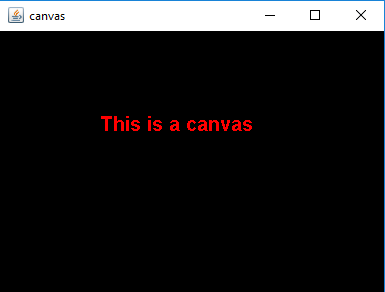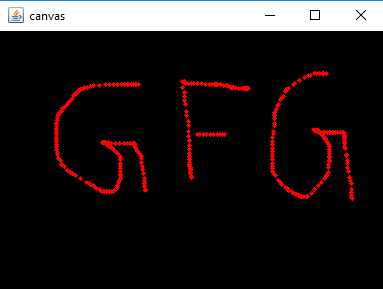# Java AWT | Canvas Class

• Last Updated : 02 Jul, 2021

Canvas class is a part of Java AWT. Canvas is a blank rectangular area where the user can draw or trap input from the user. Canvas class inherits the Component class.
Constructor of the Canvas class are :

1. Canvas(): Creates a new blank canvas.
2. Canvas(GraphicsConfiguration c): Creates a new canvas with a specified graphics configuration.

Attention reader! Don’t stop learning now. Get hold of all the important Java Foundation and Collections concepts with the Fundamentals of Java and Java Collections Course at a student-friendly price and become industry ready. To complete your preparation from learning a language to DS Algo and many more,  please refer Complete Interview Preparation Course.

Commonly used Methods in Canvas Class

Below programs illustrate the use of Canvas Class :

• Program 1: To create a canvas and paint the canvas.

## Java

 `// Java Program to create a to create``// a canvas and paint the canvas``import` `java.awt.*;``import` `javax.swing.*;``class` `canvas ``extends` `JFrame {` `    ``// constructor``    ``canvas()``    ``{``        ``super``(``"canvas"``);` `        ``// create a empty canvas``        ``Canvas c = ``new` `Canvas() {` `            ``// paint the canvas``            ``public` `void` `paint(Graphics g)``            ``{``                ``// set color to red``                ``g.setColor(Color.red);` `                ``// set Font``                ``g.setFont(``new` `Font(``"Bold"``, ``1``, ``20``));` `                ``// draw a string``                ``g.drawString(``"This is a canvas"``, ``100``, ``100``);``            ``}``        ``};` `        ``// set background``        ``c.setBackground(Color.black);` `        ``add(c);``        ``setSize(``400``, ``300``);``        ``show();``    ``}` `    ``// Main Method``    ``public` `static` `void` `main(String args[])``    ``{``        ``canvas c = ``new` `canvas();``    ``}``}`
• Output:•
• Program 2: To create a canvas and add mouse listener to the canvas(a circle of radius 5 will appear at the points where mouse are clicked or dragged on the canvas).

## Java

 `// Java Program to create a``// canvas and mouse listener to the``// canvas ( a circle of radius 5 will appear``// at the points where mouse are clicked or``//  dragged on the canvas)``import` `java.awt.*;``import` `javax.swing.*;``import` `java.awt.event.*;` `class` `canvas ``extends` `JFrame ``implements` `MouseListener, MouseMotionListener {` `    ``// create a canvas``    ``Canvas c;` `    ``// constructor``    ``canvas()``    ``{``        ``super``(``"canvas"``);` `        ``// create a empty canvas``        ``c = ``new` `Canvas() {``            ``public` `void` `paint(Graphics g)``            ``{``            ``}``        ``};` `        ``// set background``        ``c.setBackground(Color.black);` `        ``// add mouse listener``        ``c.addMouseListener(``this``);``        ``c.addMouseMotionListener(``this``);` `        ``add(c);``        ``setSize(``400``, ``300``);``        ``show();``    ``}` `    ``// mouse listener  and mouse motion listener methods``    ``public` `void` `mouseClicked(MouseEvent e)``    ``{``        ``Graphics g = c.getGraphics();` `        ``g.setColor(Color.red);` `        ``// get X and y position``        ``int` `x, y;``        ``x = e.getX();``        ``y = e.getY();` `        ``// draw a Oval at the point``        ``// where mouse is moved``        ``g.fillOval(x, y, ``5``, ``5``);``    ``}` `    ``public` `void` `mouseMoved(MouseEvent e)``    ``{``    ``}` `    ``public` `void` `mouseDragged(MouseEvent e)``    ``{``        ``Graphics g = c.getGraphics();` `        ``g.setColor(Color.red);` `        ``// get X and y position``        ``int` `x, y;``        ``x = e.getX();``        ``y = e.getY();` `        ``// draw a Oval at the point where mouse is moved``        ``g.fillOval(x, y, ``5``, ``5``);``    ``}` `    ``public` `void` `mouseExited(MouseEvent e)``    ``{``    ``}` `    ``public` `void` `mouseEntered(MouseEvent e)``    ``{``    ``}` `    ``public` `void` `mouseReleased(MouseEvent e)``    ``{``    ``}` `    ``public` `void` `mousePressed(MouseEvent e)``    ``{``    ``}``    ``// main class``    ``public` `static` `void` `main(String args[])``    ``{``        ``canvas c = ``new` `canvas();``    ``}``}`
• Output:•

My Personal Notes arrow_drop_up